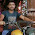Thursday, November 28, 2013

Integer constant pool in java

In this post, we'll look into Integer Wrapper class and its Integer constant pool concept with reason behind it for creating such pool with few examples.
What is Integer Class?
The Integer class wraps a value of the primitive type int in an object. An object of type Integer contains a single field whose type is int.

In addition, this class provides several methods for converting an int to a String and a String to an int, as well as other constants and methods useful when dealing with an int.

Before moving further please guess the output of the following program. If you are able to answer them all correct, then it's means you know how Java Integer Constant pool works :)

You can find the answer at the bottom of the post. If your answers are not right, then read further to know why Integer class behave in a unusual way.

Integer i = 10 vs Integer j = new Integer(10)
After compiling this class, I tried to de-compile the same class. What is got it this
So java called Integer.valueOf() whenever we create object of Integer class as Integer i = 10;

Integer.valueOf()
Let's see what does this function do. As per the Oracle doc, it says
public static Integer valueOf(int i)
Returns an Integer instance representing the specified int value.

If a new Integer instance is not required, this method should generally be used in preference to the constructor Integer(int), as this method is likely to yield significantly better space and time performance by caching frequently requested values. This method will always cache values in the range -128 to 127, inclusive, and may cache other values outside of this range.

To explore further, let's have a look at the code of Integer.valueOf() function

It's clear from the above code that when we call valueof() function with value range from -128 to 127, it always cache the value.

Integer.cache is the class that help us in caching in Integer values. The size of the cache may be controlled by the
-XX:AutoBoxCacheMax= <size>
Or this can achieved with system property
-Djava.lang.Integer.IntegerCache.high=<size>

In other words, when we create object of Integer class
Integer i = 10;
Integer j = new Integer(10);
both refer to the same instance in the pool.
So you get the same reference if value is between -128 to 127 and you call valueOf() else it just returns new Integer(int). As reference is same your == operator works for integer returned by valueOf() between this range.

Java caches the integer objects in the range -128 to 127. So, when you try to assign a value in this range to a wrapper object, the boxing operation will invoke Integer.valueOf() method and in turn it will assign a reference to the object already in the pool.
On the other hand, if you assign a value outside this range to a wrapper reference type, Integer.valueOf will create a new Integer object for that value. And hence, comparing the reference for Integer objects having value outside this range will give you false.

I hope at this point, you got the idea of Integer Constant pool. So have a look at the above code for which you have to guess the output and see this time, will you able to answer all the question correct.

Summary of Integer constant pool

IntegerClassExampleOne
i==j is not equal

IntegerClassExampleTwo
i==j is not equal

IntegerClassExampleThree
i==j is not equal

IntegerClassExampleFour
i==j is equal

If you know anyone who has started learning java, why not help them out! Just share this post with them. Thanks for studying today!...

1.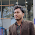Good examples have been taken, but explanation of Integer caching is not that much clear.

2.Thanks..very useful article.

3.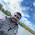nice explanation

4.great work keep doing it:)

5.This comment has been removed by the author.

6.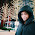Why pooling has been done for range -128 to 127 only??

1.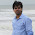That's the default range as per the Integer class code, as I mentioned this value can be configured -XX:AutoBoxCacheMax and -Djava.lang.Integer.IntegerCache.high

7.i've one question.

int a=10;
Integer b=10;

System.out.println( "a==b:" + (a==b) ); //Output: a==b:true
why it prints ture?
pls explain ?

1.Because both value are equal. why you are surprised with true? Why It should be false according to you?

2.i m confused wit int a and Integer b declarations.
can you pls tell me the diff between these two statements
int a=10;
Integer a=10;
Thank u .

8.I am technology Enthusiast. Your blog is really awesome, attractive and impressive. I like the way you think. it is very useful for Java SE & Java EE Learners. Your article adds best knowledge to our Java Online Training in India. or learn thru Java Online Training in India Students. or learn thru JavaScript Online Training in India. Appreciating the persistence you put into your blog and detailed information you provide. Kindly keep blogging.

9.